• 时间:
• 浏览:

# 算法介绍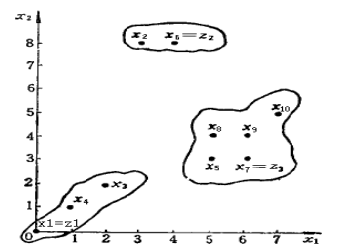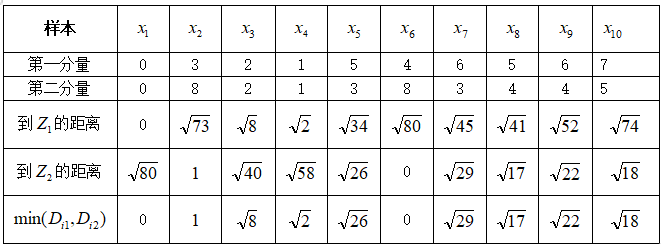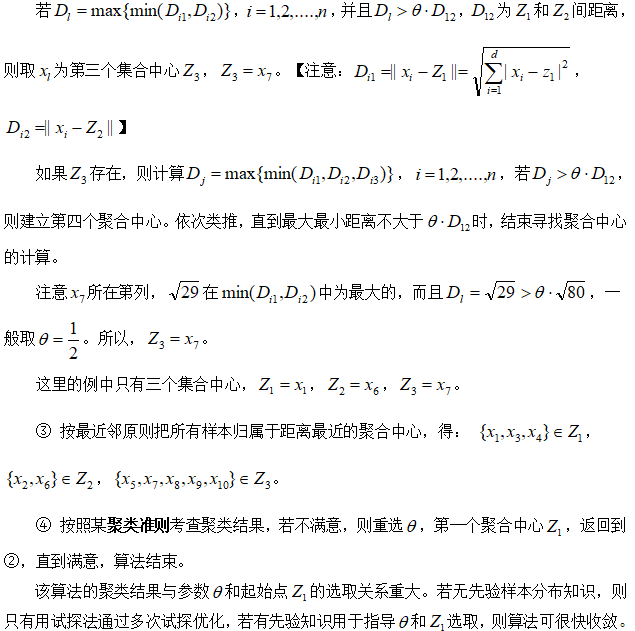# 实验内容

见图所示，为二维点集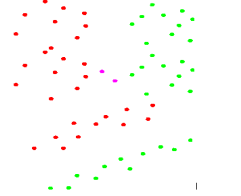# 实验步骤

1、提取分类特征，确定特征值值域，确定特征空间；

2、编写聚类程序；

3、将所提取的样本的加以聚类；

4、用误差平方和准则（也可选用其他准则）加以评价，直到满意为止。

# 代码实现 （Matlab）

clear all
clc
x=[0,0; 3,8; 2,2;1,1; 5,3; 4,8; 6,3; 5,4;  6,4;  7,5]
Theta=0.5;
[pattern,centerIndex]=MaxMinDisFun(x,0.5)
%%%%%%%%%%%%%%%%%
%函数名称 MaxMinDisFun(x,Theta)
%输入参数：
%           x  ： x为n*m的特征样本矩阵，每行为一个样本，每列为样本的特征
%         Theta：即θ，可用试探法取一固定分数，如：1/2
%输出参数：
%       pattern：输出聚类分析后的样本类别
%   centerIndex：聚类中心点
%函数功能 ：利用最大最小距离算法聚类样本数据，
%%%%%%%%%%%%%%%%%%%%%
function [classes,centerIndex]=MaxMinDisFun(x,Theta)
maxDistance=0;
start=1;    %初始选一个中心点
index=start;%相当于指针指示新中心点的位置
k=1;        %中心点计数，也即是类别
dataNum=size(x,1);  %输入的样本数
centerIndex=zeros(dataNum,1); %保存中心点
distance=zeros(dataNum,1);    %表示所有样本到当前聚类中心的距离
minDistance=zeros(dataNum,1); %取较小距离
classes=zeros(dataNum,1);     %表示类别

centerIndex(1)=index;%保存第一个聚类中心
classes(:)=k;        %初始类别全为k
%%
for i=1:dataNum
distance(i)=sqrt((x(i,:)-x(centerIndex(1),:))*(x(i,:)-x(centerIndex(1),:))');%欧氏距离，与第1个聚类中心的距离
classes(i)=k;%第1类
if(maxDistance<distance(i))
maxDistance=distance(i);%与第一个聚类中心的最大距离
index=i;%与第一个聚类中心距离最大的样本
end
end
%%
minDistance=distance;
% minDistance(index,1)=0;
maxVal=maxDistance;
while(maxVal>(maxDistance*Theta))%判断新的聚类中心是否满足条件
k=k+1;
centerIndex(k)=index;%判断新的聚类中心是否满足条件,若满足则新增聚类中心
for i=1:dataNum
distance(i)=sqrt((x(i,:)-x(centerIndex(k),:))*(x(i,:)-x(centerIndex(k),:))');%与第k个聚类中心的距离
if(minDistance(i)>distance(i))
minDistance(i)=distance(i);
classes(i)=k;%按照当前最近临方式分类，哪个近就分哪个类别
end
end
%查找minDistance中最大值
maxVal=0;
for i=1:dataNum
if((maxVal<minDistance(i)))
maxVal=minDistance(i);
index=i;
end
end

%     centerIndex(k+1)=index;%新的聚类中心
aaa=0;
end
end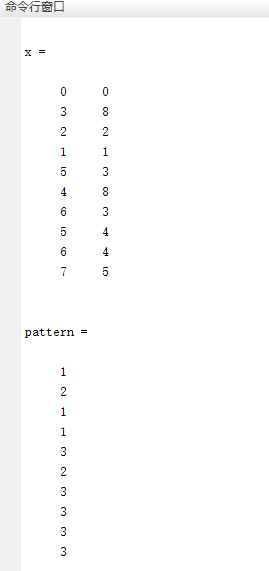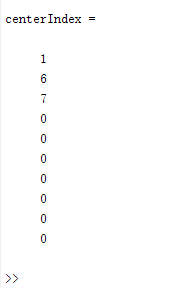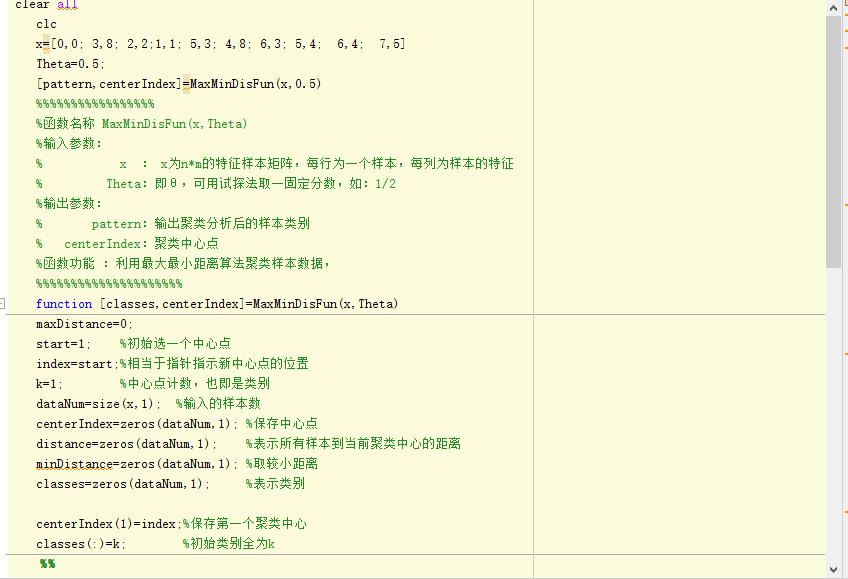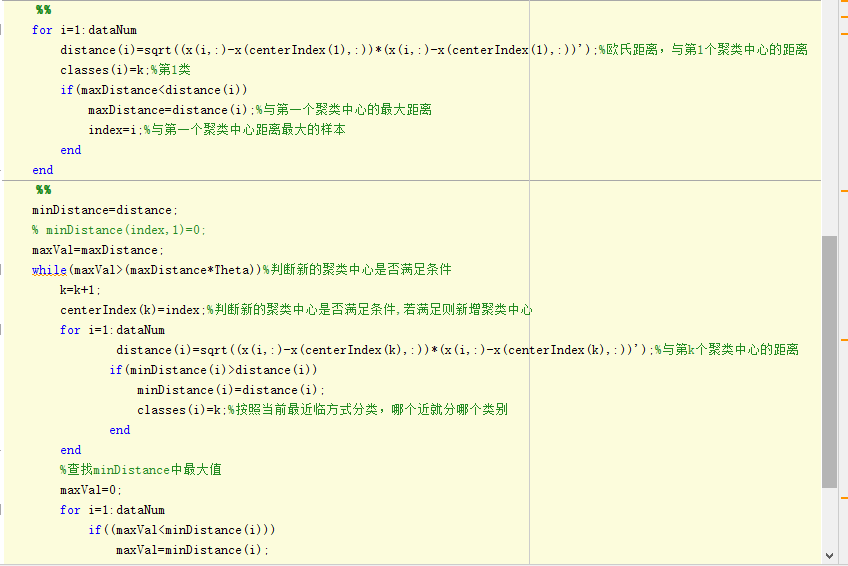中途容易犯一些小错误，反复尝试几次就可以了。